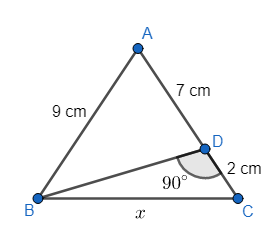Courses
Courses for Kids
Free study material
Offline Centres
MoreLast updated date: 03rd Dec 2023
Total views: 279.6k
Views today: 8.79k

# In the following figure of an isosceles triangle, find the value of $x$.(a) 6 cm(b) 8 cm(c) 4 cm(d) 2 cmVerified
279.6k+ views
Hint: We know that, in a right triangle, the square of length of hypotenuse is equal to the sum of squares of the other two edges. Using this theorem, we can first find the length of side BD. And then, again by using the same theorem, we can find the value of $x$.

Complete step-by-step solution:
We have the following figure.We can clearly see in the given figure that AB = AC, and it is also given that the triangle is isosceles.
Also, we can see that the measure of $\angle BDC={{90}^{\circ }}$.
We all know very well that the angles along a straight line is always equal to ${{180}^{\circ }}$.
Hence, we can say that $\angle BDC+\angle BDA={{180}^{\circ }}$.
We know that $\angle BDC={{90}^{\circ }}$. And so, we can say that
$\angle BDA={{180}^{\circ }}-{{90}^{\circ }}={{90}^{\circ }}$.
So, in triangle ABD, we can use the Pythagoras theorem. We have,
${{\left( AB \right)}^{2}}={{\left( BD \right)}^{2}}+{{\left( AD \right)}^{2}}$.
We can see that AB = 9 cm and AD = 7 cm.
Thus, we get
${{\left( 9 \right)}^{2}}={{\left( BD \right)}^{2}}+{{\left( 7 \right)}^{2}}$.
On simplification, we get
${{\left( BD \right)}^{2}}=81-49$.
Hence, we now have $BD=\sqrt{32}$ cm.
Now, using Pythagoras theorem in triangle BDC, we get
${{\left( BC \right)}^{2}}={{\left( BD \right)}^{2}}+{{\left( DC \right)}^{2}}$.
We now know that BD = $\sqrt{32}$ cm and DC = 2 cm. Thus, we get
${{\left( x \right)}^{2}}={{\left( \sqrt{32} \right)}^{2}}+{{\left( 2 \right)}^{2}}$.
We can simplify the above equation, to get
${{x}^{2}}=32+4$.
And so, we can write
$x=\sqrt{36}$.
Thus, $x=6$ cm.
Hence, the value of $x$ is 6 cm.

Note: We must note that the hypotenuse is the side opposite to the right angle, and we must take care of this while using the Pythagoras theorem. Also, we must take care that the length of any side of a triangle can never be a negative value. And so, we have ignored the value $x=-6$.